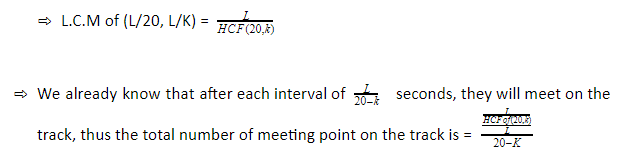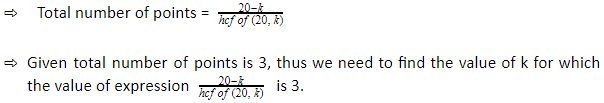# Explanation

### Video Explanation

Answer    Let the length of the track is L metre.

Relative speed of A and B is (20 – K) m/sec.

They will meet for the first time when relatively the faster person will travel the full length of the track i.e. L metre.

Thus, A and B running in the same direction will meet 1st after L/20-K seconds.

Also after each interval of L/20-K seconds, they will meet on the track.

To find the total number of meeting points on the track, we will find the number of meeting point between start time and the time when A and B again meets at starting position. As after A and B meets again at the starting position, the meeting points will start repeating.

To find the time when A and B will meet at the starting point, we find the time when A will reach the starting point individually and when B will reach the starting point individually and will take the L.C.M of both the time. [ example - if A takes 10 minutes to complete a lap and B takes 15 minutes to complete a lap, they both will meet at the starting point after 30 minutes when A has completed 3 laps and b has completed 2 laps.]

Time after which A and B will both meet at the starting point for the first time = L.C.M of (L/20, L/K).

[ L.C.M of two fraction number is the ratio of L.C.M of both numerators to H.C.F of both the denominator]. Thus,• For K = 5, 8,14, 17, the total number of meeting points is 3.

• Thus there are total 4 values of K.

Note: - Here the sides of triangle and distance of track has no significance, the number of meeting points depends on the value or ratio of speed of person meeting and not on the length of the track.

For better understanding on this topic, please see the kitabee video on special equation